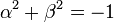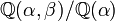# Minimal splitting field need not be contained in a cyclotomic extension of rationals

## Statement

It is possible to have a finite group$G$ and a minimal splitting field$K$ of$G$ such that$K$ has characteristic zero, but it is not contained in any cyclotomic extension of the rationals.

Note that, by the Kronecker-Weber theorem from Galois theory, being contained in a cyclotomic extension of the rationals is equivalent to being an abelian extension of the rationals, so this is equivalent to saying that it is possible for a finite group to have a minimal splitting field that is not an abelian extension of the rationals.

## Proof

### Algebraic example

Consider the quaternion group. Apart from the two-dimensional faithful irreducible representation of quaternion group, all other representations can be realized over the rationals, so a field in characteristic zero is a splitting field iff the two-dimensional faithful irreducible representation can be realized over it.

A sufficient condition for being a splitting field is that the field contain elements$\alpha,\beta$ such that$\alpha^2 + \beta^2 = -1$. We construct one such field and then argue that no proper subfield of it is a splitting field.

#### Field description

Consider the field where$\mathbb{Q}(\alpha,\beta)$ where$\alpha$ is the real cube root of 2 and$\beta$ is a square root of$-1 - \alpha^2$.

#### Proof that this field is a minimal splitting field for the quaternion group

We have$\mathbb{Q} \subseteq \mathbb{Q}(\alpha) \subseteq \mathbb{Q}(\alpha,\beta)$. The extension degree$\mathbb{Q}(\alpha)/\mathbb{Q}$ is 3 and the extension degree$\mathbb{Q}(\alpha,\beta)/\mathbb{Q}(\alpha)$ is 2 (note that the latter extension is strict because$\mathbb{Q}(\alpha)$ can be viewed as a subfield of$\R$ but$\mathbb{Q}(\alpha,\beta)$ cannot). Overall, the extension$\mathbb{Q}(\alpha,\beta)/\mathbb{Q}$ has degree$3 \times 2 = 6$.

As noted above, the field is a splitting field for the quaternion group.

Thus, it suffices to show that it contains no proper subfield that is also a splitting field for the quaternion group. For this, note that any proper subfield which is degree three over the rationals cannot work as it is formally real, and there are no proper subfields that are degree two over the rationals.

#### Proof that the field is not contained in a cyclotomic extension of the rationals

Any cyclotomic extension of the rationals has an abelian Galois group, so any subfield of it must also be a Galois extension. However,$\mathbb{Q}(\alpha) = \mathbb{Q}(2^{1/3})$ is not Galois, because the automorphism mapping$2^{1/3}$ to a complex cube root of 2 does not preserve the subfield. Therefore$\mathbb{Q}(\alpha, \beta)$ is also not contained in a cyclotomic extension of the rationals.

For this part of the proof, see also this Math StackExchange question.